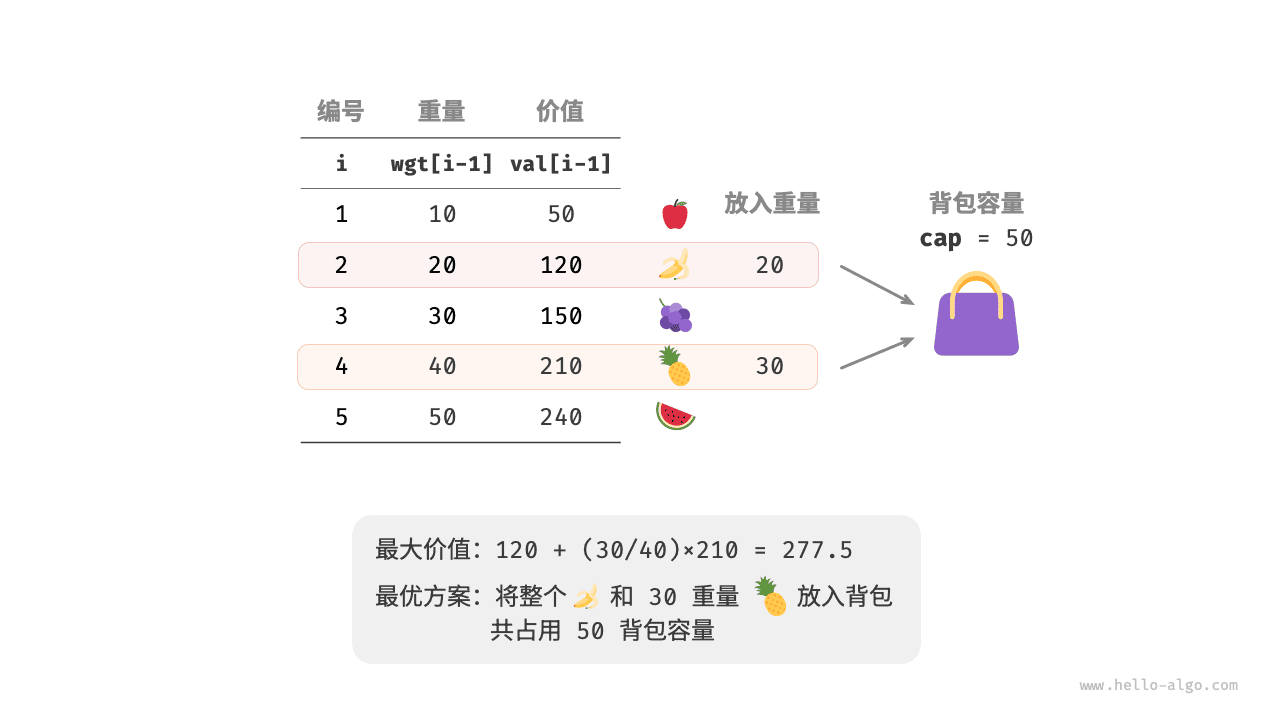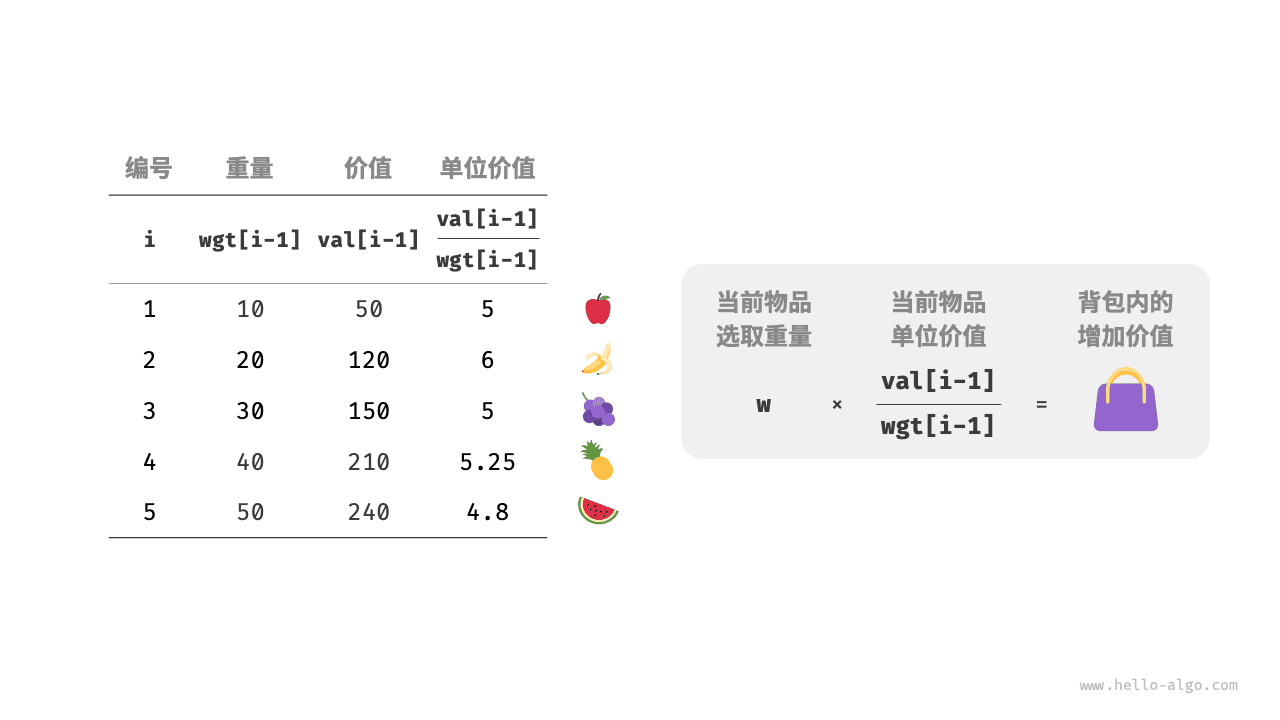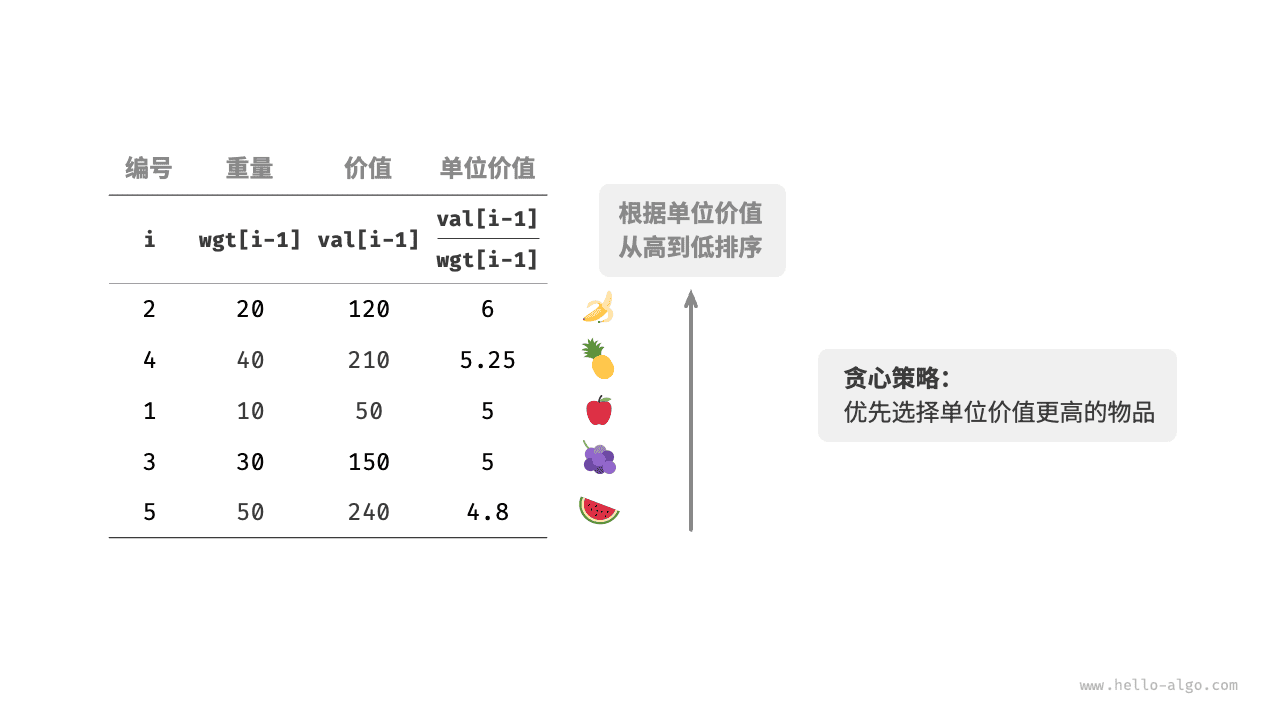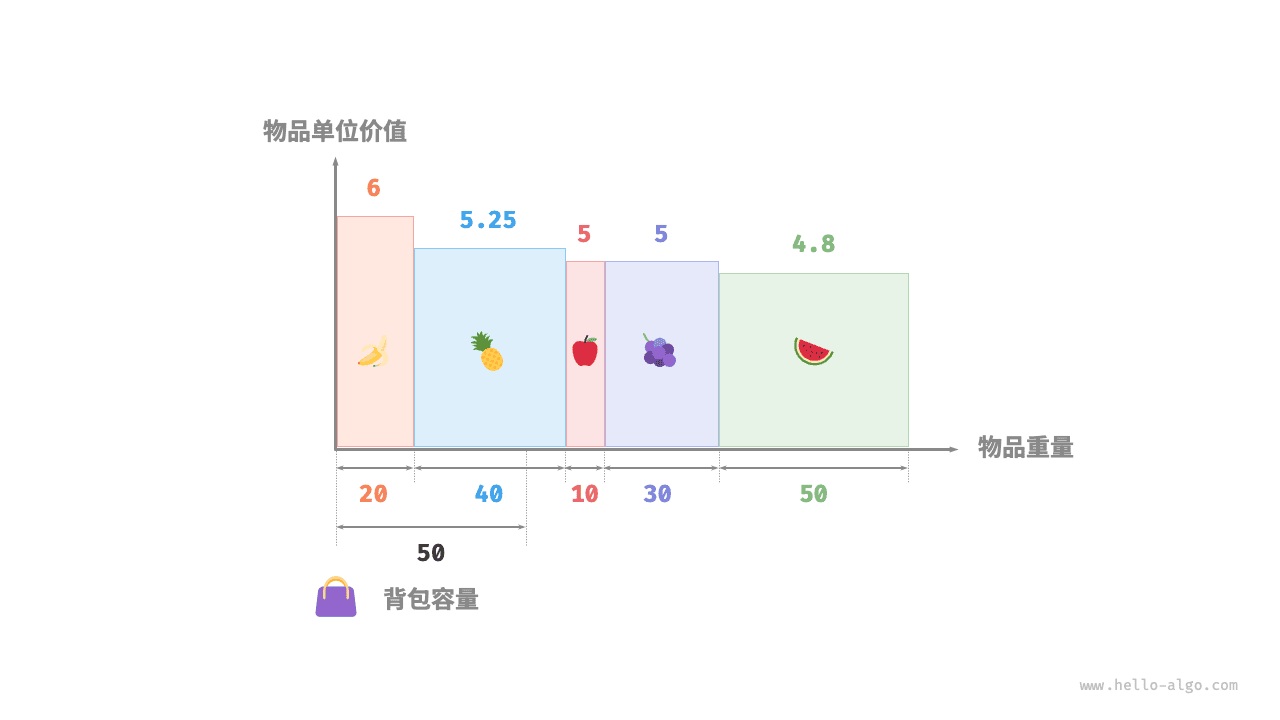# 15.2   分数背包问题¶

Question1. 对于物品 $$i$$ ，它在单位重量下的价值为 $$val[i-1] / wgt[i-1]$$ ，简称单位价值。
2. 假设放入一部分物品 $$i$$ ，重量为 $$w$$ ，则背包增加的价值为 $$w \times val[i-1] / wgt[i-1]$$### 1.   贪心策略确定¶

1. 将物品按照单位价值从高到低进行排序。
2. 遍历所有物品，每轮贪心地选择单位价值最高的物品
3. 若剩余背包容量不足，则使用当前物品的一部分填满背包。### 2.   代码实现¶

fractional_knapsack.py
class Item:
"""物品"""

def __init__(self, w: int, v: int):
self.w = w  # 物品重量
self.v = v  # 物品价值

def fractional_knapsack(wgt: list[int], val: list[int], cap: int) -> int:
"""分数背包：贪心"""
# 创建物品列表，包含两个属性：重量、价值
items = [Item(w, v) for w, v in zip(wgt, val)]
# 按照单位价值 item.v / item.w 从高到低进行排序
items.sort(key=lambda item: item.v / item.w, reverse=True)
# 循环贪心选择
res = 0
for item in items:
if item.w <= cap:
# 若剩余容量充足，则将当前物品整个装进背包
res += item.v
cap -= item.w
else:
# 若剩余容量不足，则将当前物品的一部分装进背包
res += (item.v / item.w) * cap
# 已无剩余容量，因此跳出循环
break
return res

fractional_knapsack.cpp
/* 物品 */
class Item {
public:
int w; // 物品重量
int v; // 物品价值

Item(int w, int v) : w(w), v(v) {
}
};

/* 分数背包：贪心 */
double fractionalKnapsack(vector<int> &wgt, vector<int> &val, int cap) {
// 创建物品列表，包含两个属性：重量、价值
vector<Item> items;
for (int i = 0; i < wgt.size(); i++) {
items.push_back(Item(wgt[i], val[i]));
}
// 按照单位价值 item.v / item.w 从高到低进行排序
sort(items.begin(), items.end(), [](Item &a, Item &b) { return (double)a.v / a.w > (double)b.v / b.w; });
// 循环贪心选择
double res = 0;
for (auto &item : items) {
if (item.w <= cap) {
// 若剩余容量充足，则将当前物品整个装进背包
res += item.v;
cap -= item.w;
} else {
// 若剩余容量不足，则将当前物品的一部分装进背包
res += (double)item.v / item.w * cap;
// 已无剩余容量，因此跳出循环
break;
}
}
return res;
}

fractional_knapsack.java
/* 物品 */
class Item {
int w; // 物品重量
int v; // 物品价值

public Item(int w, int v) {
this.w = w;
this.v = v;
}
}

/* 分数背包：贪心 */
double fractionalKnapsack(int[] wgt, int[] val, int cap) {
// 创建物品列表，包含两个属性：重量、价值
Item[] items = new Item[wgt.length];
for (int i = 0; i < wgt.length; i++) {
items[i] = new Item(wgt[i], val[i]);
}
// 按照单位价值 item.v / item.w 从高到低进行排序
Arrays.sort(items, Comparator.comparingDouble(item -> -((double) item.v / item.w)));
// 循环贪心选择
double res = 0;
for (Item item : items) {
if (item.w <= cap) {
// 若剩余容量充足，则将当前物品整个装进背包
res += item.v;
cap -= item.w;
} else {
// 若剩余容量不足，则将当前物品的一部分装进背包
res += (double) item.v / item.w * cap;
// 已无剩余容量，因此跳出循环
break;
}
}
return res;
}

fractional_knapsack.cs
/* 物品 */
class Item(int w, int v) {
public int w = w; // 物品重量
public int v = v; // 物品价值
}

/* 分数背包：贪心 */
double FractionalKnapsack(int[] wgt, int[] val, int cap) {
// 创建物品列表，包含两个属性：重量、价值
Item[] items = new Item[wgt.Length];
for (int i = 0; i < wgt.Length; i++) {
items[i] = new Item(wgt[i], val[i]);
}
// 按照单位价值 item.v / item.w 从高到低进行排序
Array.Sort(items, (x, y) => (y.v / y.w).CompareTo(x.v / x.w));
// 循环贪心选择
double res = 0;
foreach (Item item in items) {
if (item.w <= cap) {
// 若剩余容量充足，则将当前物品整个装进背包
res += item.v;
cap -= item.w;
} else {
// 若剩余容量不足，则将当前物品的一部分装进背包
res += (double)item.v / item.w * cap;
// 已无剩余容量，因此跳出循环
break;
}
}
return res;
}

fractional_knapsack.go
/* 物品 */
type Item struct {
w int // 物品重量
v int // 物品价值
}

/* 分数背包：贪心 */
func fractionalKnapsack(wgt []int, val []int, cap int) float64 {
// 创建物品列表，包含两个属性：重量、价值
items := make([]Item, len(wgt))
for i := 0; i < len(wgt); i++ {
items[i] = Item{wgt[i], val[i]}
}
// 按照单位价值 item.v / item.w 从高到低进行排序
sort.Slice(items, func(i, j int) bool {
return float64(items[i].v)/float64(items[i].w) > float64(items[j].v)/float64(items[j].w)
})
// 循环贪心选择
res := 0.0
for _, item := range items {
if item.w <= cap {
// 若剩余容量充足，则将当前物品整个装进背包
res += float64(item.v)
cap -= item.w
} else {
// 若剩余容量不足，则将当前物品的一部分装进背包
res += float64(item.v) / float64(item.w) * float64(cap)
// 已无剩余容量，因此跳出循环
break
}
}
return res
}

fractional_knapsack.swift
/* 物品 */
class Item {
var w: Int // 物品重量
var v: Int // 物品价值

init(w: Int, v: Int) {
self.w = w
self.v = v
}
}

/* 分数背包：贪心 */
func fractionalKnapsack(wgt: [Int], val: [Int], cap: Int) -> Double {
// 创建物品列表，包含两个属性：重量、价值
var items = zip(wgt, val).map { Item(w: $0, v:$1) }
// 按照单位价值 item.v / item.w 从高到低进行排序
items.sort(by: { -(Double($0.v) / Double($0.w)) < -(Double($1.v) / Double($1.w)) })
// 循环贪心选择
var res = 0.0
var cap = cap
for item in items {
if item.w <= cap {
// 若剩余容量充足，则将当前物品整个装进背包
res += Double(item.v)
cap -= item.w
} else {
// 若剩余容量不足，则将当前物品的一部分装进背包
res += Double(item.v) / Double(item.w) * Double(cap)
// 已无剩余容量，因此跳出循环
break
}
}
return res
}

fractional_knapsack.js
/* 物品 */
class Item {
constructor(w, v) {
this.w = w; // 物品重量
this.v = v; // 物品价值
}
}

/* 分数背包：贪心 */
function fractionalKnapsack(wgt, val, cap) {
// 创建物品列表，包含两个属性：重量、价值
const items = wgt.map((w, i) => new Item(w, val[i]));
// 按照单位价值 item.v / item.w 从高到低进行排序
items.sort((a, b) => b.v / b.w - a.v / a.w);
// 循环贪心选择
let res = 0;
for (const item of items) {
if (item.w <= cap) {
// 若剩余容量充足，则将当前物品整个装进背包
res += item.v;
cap -= item.w;
} else {
// 若剩余容量不足，则将当前物品的一部分装进背包
res += (item.v / item.w) * cap;
// 已无剩余容量，因此跳出循环
break;
}
}
return res;
}

fractional_knapsack.ts
/* 物品 */
class Item {
w: number; // 物品重量
v: number; // 物品价值

constructor(w: number, v: number) {
this.w = w;
this.v = v;
}
}

/* 分数背包：贪心 */
function fractionalKnapsack(wgt: number[], val: number[], cap: number): number {
// 创建物品列表，包含两个属性：重量、价值
const items: Item[] = wgt.map((w, i) => new Item(w, val[i]));
// 按照单位价值 item.v / item.w 从高到低进行排序
items.sort((a, b) => b.v / b.w - a.v / a.w);
// 循环贪心选择
let res = 0;
for (const item of items) {
if (item.w <= cap) {
// 若剩余容量充足，则将当前物品整个装进背包
res += item.v;
cap -= item.w;
} else {
// 若剩余容量不足，则将当前物品的一部分装进背包
res += (item.v / item.w) * cap;
// 已无剩余容量，因此跳出循环
break;
}
}
return res;
}

fractional_knapsack.dart
/* 物品 */
class Item {
int w; // 物品重量
int v; // 物品价值

Item(this.w, this.v);
}

/* 分数背包：贪心 */
double fractionalKnapsack(List<int> wgt, List<int> val, int cap) {
// 创建物品列表，包含两个属性：重量、价值
List<Item> items = List.generate(wgt.length, (i) => Item(wgt[i], val[i]));
// 按照单位价值 item.v / item.w 从高到低进行排序
items.sort((a, b) => (b.v / b.w).compareTo(a.v / a.w));
// 循环贪心选择
double res = 0;
for (Item item in items) {
if (item.w <= cap) {
// 若剩余容量充足，则将当前物品整个装进背包
res += item.v;
cap -= item.w;
} else {
// 若剩余容量不足，则将当前物品的一部分装进背包
res += item.v / item.w * cap;
// 已无剩余容量，因此跳出循环
break;
}
}
return res;
}

fractional_knapsack.rs
/* 物品 */
struct Item {
w: i32, // 物品重量
v: i32, // 物品价值
}

impl Item {
fn new(w: i32, v: i32) -> Self {
Self { w, v }
}
}

/* 分数背包：贪心 */
fn fractional_knapsack(wgt: &[i32], val: &[i32], mut cap: i32) -> f64 {
// 创建物品列表，包含两个属性：重量、价值
let mut items = wgt
.iter()
.zip(val.iter())
.map(|(&w, &v)| Item::new(w, v))
.collect::<Vec<Item>>();
// 按照单位价值 item.v / item.w 从高到低进行排序
items.sort_by(|a, b| {
(b.v as f64 / b.w as f64)
.partial_cmp(&(a.v as f64 / a.w as f64))
.unwrap()
});
// 循环贪心选择
let mut res = 0.0;
for item in &items {
if item.w <= cap {
// 若剩余容量充足，则将当前物品整个装进背包
res += item.v as f64;
cap -= item.w;
} else {
// 若剩余容量不足，则将当前物品的一部分装进背包
res += item.v as f64 / item.w as f64 * cap as f64;
// 已无剩余容量，因此跳出循环
break;
}
}
res
}

fractional_knapsack.c
/* 物品 */
typedef struct {
int w; // 物品重量
int v; // 物品价值
} Item;

/* 分数背包：贪心 */
float fractionalKnapsack(int wgt[], int val[], int itemCount, int cap) {
// 创建物品列表，包含两个属性：重量、价值
Item *items = malloc(sizeof(Item) * itemCount);
for (int i = 0; i < itemCount; i++) {
items[i] = (Item){.w = wgt[i], .v = val[i]};
}
// 按照单位价值 item.v / item.w 从高到低进行排序
qsort(items, (size_t)itemCount, sizeof(Item), sortByValueDensity);
// 循环贪心选择
float res = 0.0;
for (int i = 0; i < itemCount; i++) {
if (items[i].w <= cap) {
// 若剩余容量充足，则将当前物品整个装进背包
res += items[i].v;
cap -= items[i].w;
} else {
// 若剩余容量不足，则将当前物品的一部分装进背包
res += (float)cap / items[i].w * items[i].v;
cap = 0;
break;
}
}
free(items);
return res;
}

fractional_knapsack.zig
[class]{Item}-[func]{}

[class]{}-[func]{fractionalKnapsack}


### 3.   正确性证明¶